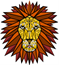# Tauchain (TML) - Partial Fixed Point Logic and it's unique benefits

3년 전

## What is partial fixed point logic?

Partial fixed point logic is a first order logic. First order logic is also known as predicate calculus with a flow like "if x..then y..." or "There exists x such that x is Socrates and X is a man". First order logic is very similar to propositional logic but has the addition of a quantifier. Partial fixed point rewards TML with the benefits of being both sound and compete.

Soundness is among the most fundamental properties of mathematical logic. The soundness property provides the initial reason for counting a logical system as desirable. The completeness property means that every validity (truth) is provable. Together they imply that all and only validities are provable.

Clarification

First order logic is typically undecidable, so the validity of my previous statement cannot be guaranteed. For the clearest possible understanding, watch this lecture by professor Dawar:

Decidable Fragments of First-Order and Fixed-Point Logic discusses this and we also know from Church and Turing that first order logic is undecidable.

## Datalog as an example of a programming language using first order logic

If we take a look at datalog we can get an idea of what capabilities Tau Meta Language under partial fixed point logic must have. Datalog is a deductive database language which is a synatic subset of Prolog. Datalog which is based on least fixed point logic is considered a domain specific language because it is NOT Turing Complete. It is a language used primarily for Query Resolution purposes. Yet Query Evaluation is sound and complete with Datalog, and so we can at least get a good idea of what that means by looking at Datalog even though TML takes things a step further.

## Conclusion

• Partial fixed point logic is first order logic.
• This logic is similar to what we see from logic behind the language called Datalog.
• In that using this logic we can create a language which on finite rather than infinite sets is guaranteed to terminate (no halting problem) on finite machines.
• Not Turing complete.
• Due to it being based on first order logic and specifically if based on partial fixed point first order logic, then we get the rewards of having soundness and completeness.

So we now know the features Tau Meta Language using partial fixed point logic will have. We know that this TML being multi-logic can give us the security guarantees not available anywhere else (not Ethereum, Tezos, or EOS). We know also that partial fixed point logic is P-SPACE based on an understanding of "descriptive complexity". In specific, FO is the complexity class associated with formulas of first order logic and so this complexity class is relevant to Tau Meta Language utilizing partial fixed point logic.

Restricting predicates to be from a set X yields a smaller class FO[X]. For instance, FO[<] is the set of star-free languages. The two different definitions of FO[<], in terms of logic and in terms of regular expressions, suggests that this class may be mathematically interesting beyond its role in computational complexity, and that methods from both logic and regular expressions may be useful in its study.

Datalog seems to be an example of this as it fits this profile. TauML seems to be going down this path. Finite model theory connects all the dots for those who want to aggressively dig deep into this subject because TML utilizing pfp is first order logic and finite model theory addresses this precisely.

## References

Sort Order:  trending

@dana-edwards - Ouch. This post makes me realize that I have a lot of reading to do. I feel totally outdated. Sorry - unable to offer a meaningful comment at this point in time. Will read up and revisit sometime. Thanks
Regards,@vm2904

·  3년 전

Sounds like dark matter.

·  3년 전

Too technical for me Dana , it was a bouncer :-) .
Regards Nainaz
#thealliance

·
·  3년 전

Another enlightening post I took some new things from. I'll take a closer look at Datalog and Prolog. Also thanks for the link to the talk by profesor Dawar, great stuff. Have a nice Christmas!
P.S.: Have you considered making a post about the concept of Touring completeness? I got it after emerging myself into the material, but I still struggle when explaining it to others in a meaningful manner.

·
·  3년 전

Turing completeness involves an abstract machine with infinite memory, being able to compute anything computable. The universe is finite, all apps running on computers are finite, but some algorithms you want to construct in a way which assumes the infinite because you have no way of predicting the future or when it should halt. If you know exactly how much resources the app will need then you can restrict the app to that amount, and it always halts.

Happy holidays.

·
·
·  3년 전

The universe is finite, all apps running on computers are finite, but some algorithms you want to construct in a way which assumes the infinite because you have no way of predicting the future or when it should halt.

This is a basic principle of FMT, and even quantum computers use a Boolean circuit model (Quantum circuit), making them Quantum Turing machines.

·
·